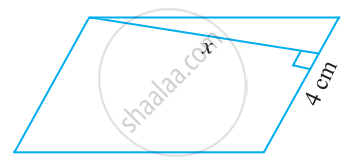# Find the height ‘x’ if the area of the parallelogram is 24 cm2 and the base is 4 cm. - Mathematics

Sum

Find the height ‘x’ if the area of the parallelogram is 24 cm2 and the base is 4 cm.#### Solution

Area of parallelogram = b × h

Therefore, 24 = 4 × x

24/4 = x

x = 6 cm

So, the height of the parallelogram is 6 cm.

Concept: Area of a Parallelogram
Is there an error in this question or solution?